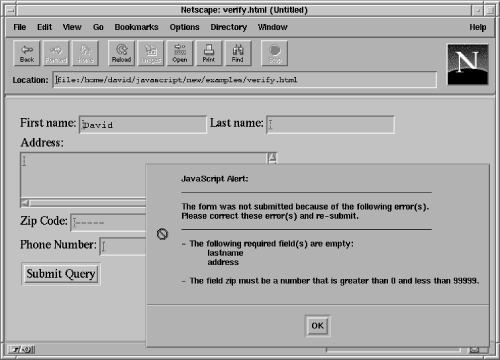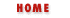#Chapter 17Forms and Form Elements## 17.6 Form Verification Example

We'll close our discussion of forms with an extended example that demonstrates several of the concepts we've been talking about. Example 17.2 shows how you might use the onSubmit() event handler of the Form object to perform input validation to notify the user and prevent the form from being submitted when it contains missing or invalid data. After studying this example, you may want to turn back to Example 1.3, the forms programming example with which we began this book. The code of that example will probably make more sense now that you are a JavaScript expert!

Example 17.2 defines a verify() function suitable for use as a generic form validator. It checks for empty non-optional fields, and can also check that numeric values are in fact numeric and that they fall within a specified numeric range. This verify() function relies on the type property of form elements to determine which elements are which, and also relies on additional user-defined properties to distinguish optional fields from required fields and to specify the allowed range for numeric fields. Note also how it reads the value property of input fields, and uses the name property of those fields when reporting errors. Figure 17.3 shows an example form using this verification scheme, and the error message that is displayed when the user attempts to submit the form before correctly filling it in.

#### Figure 17.3: A form that failed validation#### Example 17.2: Performing Form Validation

```<SCRIPT LANGUAGE="JavaScript1.1">
// A utility function that returns true if a string contains only
// whitespace characters.
function isblank(s)
{
for(var i = 0; i < s.length; i++) {
var c = s.charAt(i);
if ((c != ' ') && (c != '\n') && (c != '\t')) return false;
}
return true;
}
// This is the function that performs form verification. It will be invoked
// from the onSubmit() event handler. The handler should return whatever
// value this function returns.
function verify(f)
{
var msg;
var empty_fields = "";
var errors = "";

// Loop through the elements of the form, looking for all
// text and textarea elements that don't have an "optional" property
// defined. Then, check for fields that are empty and make a list of them.
// Also, if any of these elements have a "min" or a "max" property defined,
// then verify that they are numbers and that they are in the right range.
// Put together error messages for fields that are wrong.
for(var i = 0; i < f.length; i++) {
var e = f.elements[i];
if (((e.type == "text") || (e.type == "textarea")) && !e.optional) {
// first check if the field is empty
if ((e.value == null) || (e.value == "") || isblank(e.value)) {
empty_fields += "\n          " + e.name;
continue;
}
// Now check for fields that are supposed to be numeric.
if (e.numeric || (e.min != null) || (e.max != null)) {
var v = parseFloat(e.value);
if (isNaN(v) ||
((e.min != null) && (v < e.min)) ||
((e.max != null) && (v > e.max))) {
errors += "- The field " + e.name + " must be a number";
if (e.min != null)
errors += " that is greater than " + e.min;
if (e.max != null && e.min != null)
errors += " and less than " + e.max;
else if (e.max != null)
errors += " that is less than " + e.max;
errors += ".\n";
}
}
}
}
// Now, if there were any errors, then display the messages, and
// return false to prevent the form from being submitted. Otherwise
// return true.
if (!empty_fields && !errors) return true;
msg  = "______________________________________________________\n\n"
msg += "The form was not submitted because of the following error(s).\n";
msg += "Please correct these error(s) and re-submit.\n";
msg += "______________________________________________________\n\n"
if (empty_fields) {
msg += "- The following required field(s) are empty:"
+ empty_fields + "\n";
if (errors) msg += "\n";
}
msg += errors;
return false;
}
</SCRIPT>
<!------------------------------------------------------------------------
Here's a sample form to test our verification with. Note that we
call verify() from the onSubmit() event handler, and return whatever
value it returns. Also note that we use the onSubmit() handler as
an opportunity to set properties on the form objects that verify()
will use in the verification process.
-------------------------------------------------------------------------->
<FORM onSubmit="
this.firstname.optional = true;
this.phonenumber.optional = true;
this.zip.min = 0;
this.zip.max = 99999;
return verify(this);
">
First name: <INPUT TYPE=text NAME="firstname">
Last name: <INPUT TYPE=text NAME="lastname"><BR>Naming Forms and Form ElementsCompatibility Techniques